# AC Waveforms

## AC Electric Circuits

• #### Question 1

Radio waves are comprised of oscillating electric and magnetic fields, which radiate away from sources of high-frequency AC at (nearly) the speed of light. An important measure of a radio wave is its wavelength, defined as the distance the wave travels in one complete cycle.

Suppose a radio transmitter operates at a fixed frequency of 950 kHz. Calculate the approximate wavelength (λ) of the radio waves emanating from the transmitter tower, in the metric distance unit of meters. Also, write the equation you used to solve for λ.

• #### Question 2

What is the difference between DC and AC electricity? Identify some common sources of each type of electricity.

• #### Question 3

Alternating current produced by electromechanical generators (or alternators as they are sometimes designated) typically follows a sine-wave pattern over time. Plot a sine wave on the following graph, by tracing the height of a rotating vector inside the circle to the left of the graph: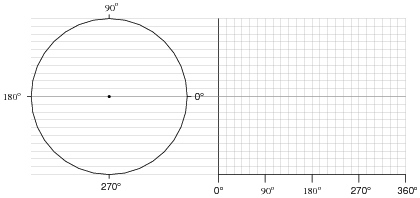To illustrate the principle here, I will show how the point is plotted for a rotation of 45o: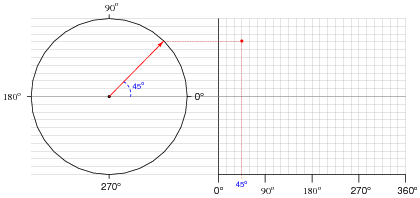You may wish to use a protractor to precisely mark the angles along the rotation of the circle, in making your sine-wave plot.

• #### Question 4

Apply the following terms to this graph of an AC voltage measured over time:

Frequency
Period
Hertz
Amplitude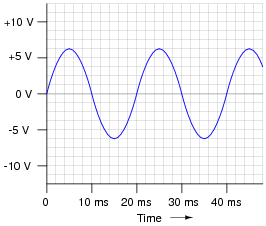• #### Question 5

Frequency used to be expressed in units of cycles per second, abbreviated as CPS. Now, the standardized unit is Hertz. Explain the meaning of the obsolete frequency unit: what, exactly, does it mean for an AC voltage or current to have x number of “cycles per second?”

• #### Question 6

If an AC voltage has a frequency of 350 Hz, how long (in time) is its period?

• #### Question 7

If the only instrument you had in your possession to detect AC voltage signals was an audio speaker, how could you use it to determine which of two AC voltage waveforms has the greatest period?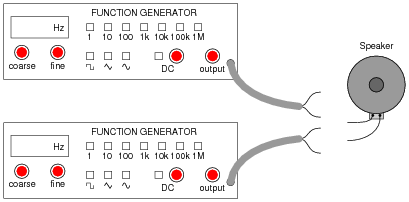• #### Question 8

Determine the frequency of a waveform having a period of 1.4 milliseconds (1.4 ms).

• #### Question 9

Alternating current produced by electromechanical generators (or alternators as they are sometimes designated) typically follows a sine-wave pattern over time. Using a calculator, or a set of “trig tables,” plot a sine wave on the following graph: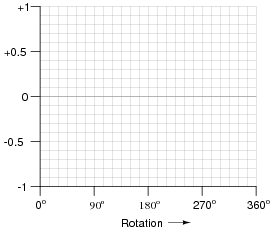• #### Question 10

Define the following terms:

Frequency
Wavelength
Cycle
Hertz
Amplitude